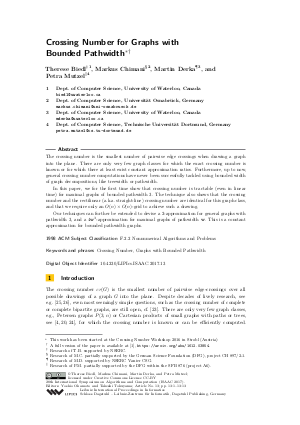Document# Crossing Number for Graphs with Bounded~Pathwidth

### Authors Therese Biedl, Markus Chimani, Martin Derka, Petra Mutzel## File

LIPIcs.ISAAC.2017.13.pdf
• Filesize: 0.58 MB
• 13 pages

## Cite As

Therese Biedl, Markus Chimani, Martin Derka, and Petra Mutzel. Crossing Number for Graphs with Bounded~Pathwidth. In 28th International Symposium on Algorithms and Computation (ISAAC 2017). Leibniz International Proceedings in Informatics (LIPIcs), Volume 92, pp. 13:1-13:13, Schloss Dagstuhl - Leibniz-Zentrum für Informatik (2017)
https://doi.org/10.4230/LIPIcs.ISAAC.2017.13

## Abstract

The crossing number is the smallest number of pairwise edge crossings when drawing a graph into the plane. There are only very few graph classes for which the exact crossing number is known or for which there at least exist constant approximation ratios. Furthermore, up to now, general crossing number computations have never been successfully tackled using bounded width of graph decompositions, like treewidth or pathwidth. In this paper, we for the first time show that crossing number is tractable (even in linear time) for maximal graphs of bounded pathwidth 3. The technique also shows that the crossing number and the rectilinear (a.k.a. straight-line) crossing number are identical for this graph class, and that we require only an O(n)xO(n)-grid to achieve such a drawing. Our techniques can further be extended to devise a 2-approximation for general graphs with pathwidth 3, and a 4w^3-approximation for maximal graphs of pathwidth w. This is a constant approximation for bounded pathwidth graphs.
##### Keywords
• Crossing Number
• Graphs with Bounded Pathwidth

## Metrics

• Access Statistics
• Total Accesses (updated on a weekly basis)
0

## References

1. T. Biedl, M. Chimani, M. Derka, and P. Mutzel. Crossing number for graphs with bounded pathwidth. CoRR, abs/1612.03854, 2016. URL: http://arxiv.org/abs/1612.03854.
2. H.L. Bodlaender. A linear-time algorithm for finding tree-decompositions of small treewidth. SIAM J. Comput., 25(6):1305-1317, 1996. URL: http://dx.doi.org/10.1137/S0097539793251219.
3. H.L. Bodlaender and T. Kloks. Efficient and constructive algorithms for the pathwidth and treewidth of graphs. J. Algorithms, 21(2):358-402, 1996. URL: http://dx.doi.org/10.1006/jagm.1996.0049.
4. D. Bokal. On the crossing numbers of cartesian products with paths. J. Comb. Theory Ser. B, 97(3):381-384, May 2007. URL: http://dx.doi.org/10.1016/j.jctb.2006.06.003.
5. S. Cabello. Hardness of approximation for crossing number. Discrete & Computational Geometry, 49(2):348-358, 2013. URL: http://dx.doi.org/10.1007/s00454-012-9440-6.
6. S. Cabello and B. Mohar. Crossing number and weighted crossing number of near-planar graphs. Algorithmica, 60(3):484-504, 2011. URL: http://dx.doi.org/10.1007/s00453-009-9357-5.
7. M. Chimani and P. Hliněný. A tighter insertion-based approximation of the crossing number. Journal of Combinatorial Optimization, pages 1-43, 2016. URL: http://dx.doi.org/10.1007/s10878-016-0030-z.
8. M. Chimani and P. Hliněný. Inserting multiple edges into a planar graph. In SoCG 2016, pages 30:1-30:15. LIPIcs, 2016. URL: http://dx.doi.org/10.4230/LIPIcs.SoCG.2016.30.
9. M. Chimani, P. Hliněný, and P. Mutzel. Vertex insertion approximates the crossing number for apex graphs. European Journal of Combinatorics, 33:326-335, 2012.10. J. Chuzhoy. An algorithm for the graph crossing number problem. In STOC '11, pages 303-312. ACM, 2011.11. B. Courcelle. The monadic second-order logic of graphs. I. Recognizable sets of finite graphs. Information and Computation, 85(1):12-75, 1990. URL: http://dx.doi.org/10.1016/0890-5401(90)90043-H.
12. J. Fox, J. Pach, and A. Suk. Approximating the rectilinear crossing number. In GD 2016, LNCS 9801, pages 413-426. Springer, 2016. URL: http://dx.doi.org/10.1007/978-3-319-50106-2_32.
13. I. Gitler, P. Hliněný, J. Leanos, and G. Salazar. The crossing number of a projective graph is quadratic in the face-width. Electronic Journal of Combinatorics, 15(1):#R46, 2008.14. M. Grohe. Computing crossing numbers in quadratic time. J. Comput. Syst. Sci., 68(2):285-302, 2004.15. P. Hliněný. Crossing-number critical graphs have bounded path-width. J. Comb. Theory, Ser. B, 88(2):347-367, 2003. URL: http://dx.doi.org/10.1016/S0095-8956(03)00037-6.
16. P. Hliněný and M. Chimani. Approximating the crossing number of graphs embeddable in any orientable surface. In SODA '10, pages 918-927, 2010.17. P. Hliněný and G. Salazar. Approximating the crossing number of toroidal graphs. In ISAAC '07, LNCS 4835, pages 148-159. Springer, 2007.18. K-I. Kawarabayashi and B. Reed. Computing crossing number in linear time. In STOC '07, pages 382-390, 2007.19. D.J. Kleitman. The crossing number of K_5,n. J. of Comb. Theory, 9(4):315-323, 1970. URL: http://dx.doi.org/10.1016/S0021-9800(70)80087-4.
20. M. Klešč and J. Petrillová. The crossing numbers of products of path with graphs of order six. Discussiones Mathematicae Graph Theory, 33(3):571-582, 2013.21. T. Kloks. Treewidth, Computations and Approximations. LNCS 842. Springer, 1994. URL: http://dx.doi.org/10.1007/BFb0045375.
22. F.T. Leighton. Complexity Issues in VLSI: Optimal Layouts for the Shuffle-exchange Graph and Other Networks. MIT Press, Cambridge, MA, USA, 1983.23. S. Pan and R.B. Richter. The crossing number of K_11 is 100. Journal of Graph Theory, 56(2):128-134, 2007. URL: http://dx.doi.org/10.1002/jgt.20249.
24. R.B. Richter and G. Salazar. The crossing number of P(N,3). Graphs and Combinatorics, 18(2):381-394, 2002. URL: http://dx.doi.org/10.1007/s003730200028.
25. M. Schaefer. The graph crossing number and its variants: A survey. Electronic Journal of Combinatorics, #DS21, May 15, 2014.26. I. Vrt'o. Crossing numbers of graphs: A bibliography. ftp://ftp.ifi.savba.sk/pub/imrich/crobib.pdf, 2014.
27. D.R. Wood and J.A. Telle. Planar decompositions and the crossing number of graphs with an excluded minor. New York J. Math., 13:117-146, 2007.X

Feedback for Dagstuhl Publishing